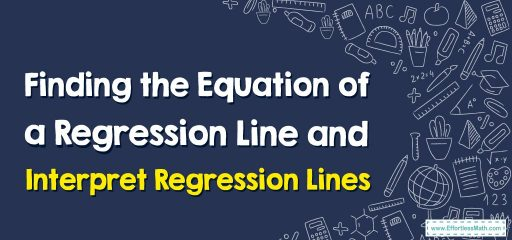# How to Find the Equation of a Regression Line and Interpret Regression LinesThe equation of the regression line is calculated using the least squares method and is the line that best fits the set of data points in the scatter plot.

Finding the regression line in several steps is fully and accurately explained in this article.

Step $$1$$: Collect data on the two desired variables and organize them in a scatter plot.

Step $$2$$: As mentioned at the beginning of the article, the equation of the line of best fit is found using the least squares method. To find the line, you must first calculate the slope ($$m$$) and $$y$$-intercept ($$b$$). Slope and $$y$$-intercept are calculated using the following formulas:

$$m=\frac{(nΣ(xy)-(Σx)(Σy))}{(nΣ(x^2)-(Σx)^2)}$$

$$b=\frac{(Σy-mΣx)}{n}$$

Step $$3$$: Using the found slope and $$y$$-intercept, the equation of the regression line will be $$y=mx+b$$.

Step $$4$$: The slope and $$y$$-intercept of the regression line are used to interpret the line. The slope shows the amount of change in $$y$$ against a unit change in $$x$$ and can be interpreted as the strength and direction of the relationship between the variables. A positive slope indicates a positive relationship, while a negative slope indicates a negative relationship. The slope shows the amount of change in $$y$$ against a unit change in $$x$$ and can be interpreted as the strength and direction of the relationship between the variables. A positive slope indicates a positive relationship, while a negative slope indicates a negative relationship. The $$y$$-intercept represents the value of $$y$$ when $$x=0$$ and represents the intersection of the line with the $$y$$-axis.

Step $$5$$: The correlation coefficient ($$r$$) is used to measure the strength and direction of the linear relationship between two variables. A correlation coefficient of $$+1$$ indicates a complete positive linear relationship, a coefficient of $$-1$$ indicates a complete negative linear relationship, and a coefficient of $$0$$ indicates the absence of a linear relationship. You can use the correlation coefficient for interpretation.

Step $$6$$: To interpret the regression line, you can also use the coefficient of determination ($$R^2$$), which indicates the ratio of changes in the dependent variable that is explained by the independent variable. $$R^2$$ is always between $$0$$ and $$1$$, and the closer $$R^2$$ is to $$1$$, the better the model explains the data.

A regression line is only an approximation of the relationship between variables and may not accurately describe the relationship for values of $$x$$ that are outside the range of the data.

### What people say about "How to Find the Equation of a Regression Line and Interpret Regression Lines - Effortless Math: We Help Students Learn to LOVE Mathematics"?

No one replied yet.

X
30% OFF

Limited time only!

Save Over 30%

SAVE $5 It was$16.99 now it is \$11.99